# How many pixels is 1 cm?

﻿

How many pixels are contained in one centimeter - it would seem, the question is obvious, there should be no dirty tricks here. But everything is not as simple as it seems at first glance. The fact is that a pixel is not a fixed value - it is the smallest logical element of a two-dimensional raster image that has its own size, transparency, coordinates, color. Therefore, we will take a closer look at these pixel properties and, to solve the problem, we will get acquainted with such concepts as the printer resolution (DPI) and monitor screen resolution (PPI).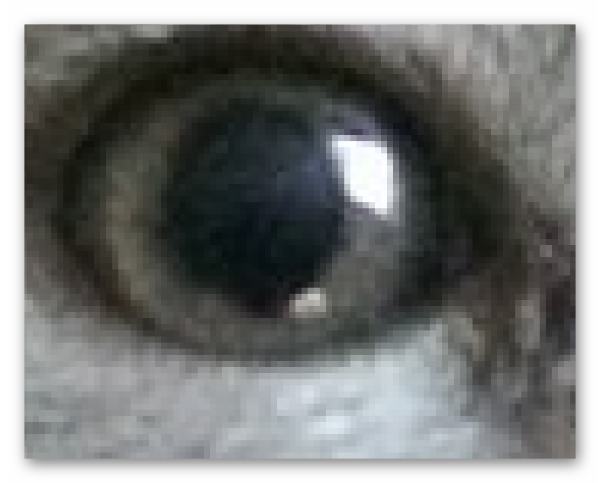This is how the image looks like under high magnification. The small squares that you can see in this photo are the very pixels.

﻿

The content of the article:

## What does the term "Permissions" mean?

The number of pixels, along with the digit of the palette, are one of the most important characteristics affecting image quality. All this needs to be known to determine the number of pixels in 1 centimeter. The smaller the pixel, the more detailed the final image will be. This is due to the fact that with a smaller pixel size, their number per unit area increases. Let's enter a value characterizing the number of pixels per unit area and call it Resolution.   This characteristic has four varieties, depending on the type of image conversion - DPI, PPI, LTI and SPI. The main points here are the DPI and PPI values, consider them in more detail.

• DPI is the number of dots per inch, type of resolution applied to printers when printing images. The larger the parameter, the more detailed the image will be when printed.
• PPI - the number of pixels per inch, is used to indicate the resolution of the monitor. This value, most often, counts the number of pixels that fit on the screen of your monitor.

Thus, if we talk about images directly, then it should be noted that it does not have its own resolution. This parameter is formed by the device on which the image was created. For example, if the photo A is taken on a 3-megapixel camera, then its resolution will be equal to 2048 pixels in width and 1536 in height. If 4-megapixel camera was used for the picture B, then, accordingly, the resolution of this image will be 2464 pixels in width and 1632 in height.

It is logical to further emphasize the relationship of screen resolution with image size. Take the examples described above. If you print the image A to print with a resolution of 300 DPI, then at the output we will get a photo with dimensions of 17 × 13 centimeters. If you print a photo B, then it will measure 19 × 14 centimeters. The same trend will be observed when displaying image data on the monitor screen. Photo B will take on the display larger sizes than photo A.

﻿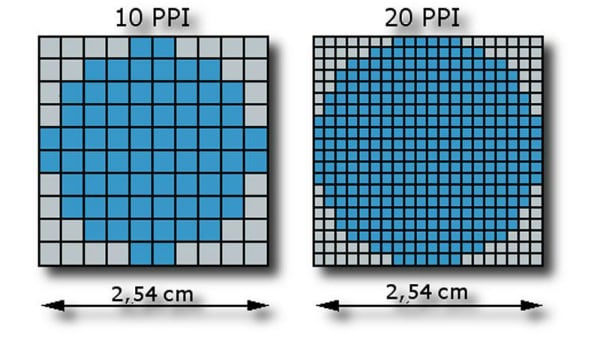Here you can see the difference in the number of pixels per unit area. As you can see, despite the same size, the image on the right will look more accurate than on the left, since the PPI of the second image is larger.

A curious conclusion follows from this - the resolution in its pure form is not a measure of the accuracy and quality of the image, it only forms the final dimensions at which the picture will have the highest detail. But, given the fact that it is more convenient for people to look at larger images, we can conditionally assign the value of this characteristic to the main one when describing the degree of detail.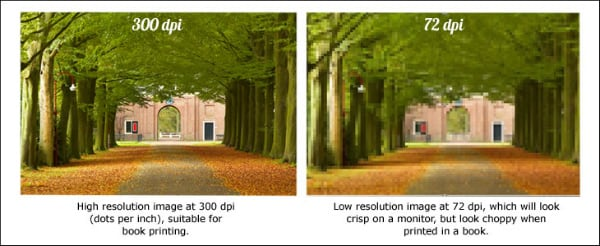Here is a vivid example showing how at the same size, but different resolution, the images will look like when printed.

It is time to get acquainted with the principle of determining the pixel size of 1 cm.

## Determining the number of pixels in 1 centimeter

Before you get acquainted with the above terms and patterns, you probably were concerned with only one question - the number of pixels in 1 cm. Now you understand that the number of pixels per unit area, that is, resolution is not a fixed value. And it depends on the size of the pixel itself; moreover, it is variable if we talk about outputting the image to a flat carrier.

﻿

Well, how to determine the size of a pixel? In fact, this question is very tricky. After all, there is no such thing as a “pixel size”. Pixel is not an independent value - it is part of the relationship between screen resolution, physical size and pixel size of a given display. Any pixel properties are set by the device in which the image is processed. But, it is precisely from here, from this definition follows the formula that allows you to determine the number of pixels per unit area, that is, the resolution of the PPI:

P / U = R , where P is the pixel size of the screen, U is the physical size of the screen, and R is the number of pixels per inch.

For example, one of Apple's Mac Cinema Display 27 screens has a physical width of 23.5 inches, its pixel width is 2560. From this data, we can calculate the pixel density per inch:

2560 / 23.5 = 109 pixels per one inch of this display. Let's try to convert this value to centimeters:

1 inch = 2.54 cm, therefore, 109 / 2.54 = 42 pixels per centimeter, so we calculated how many pixels are in one centimeter of this screen.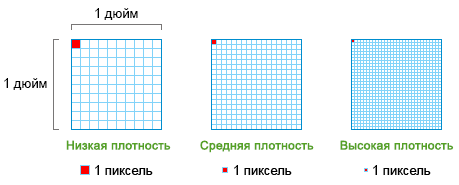The figure shows the various degrees of image density by which you can navigate in terms of creating a photo that is optimal in resolution.

### Formula # 2 for calculating PPI

There is an alternative formula for determining PPI. To do this, we need to know the screen diagonal:

[ √W ^ 2 + H ^ 2] / D = R , where W is the width of the screen in pixels, H is the height, and D is the diagonal, expressed in inches. I propose to apply this formula to the above example:

• Since the aspect ratio of Mac Cinema Display is 16: 9, and the pixel width is 2560, we can calculate the height of the display from here:

(2560/16) * 9 = 1440;

1. The screen diagonal we are considering is 27 inches;
2. Substitute these values ​​into the formula and find the pixel density per inch:

[√2560 ^ 2 + 1440 ^ 2] / 27 = 109 PPI, that is, the same 42 pixels per centimeter.

Using these formulas, you can calculate how many pixels are in one centimeter, provided that the photo is taken using a digital device, such as a camera. When printing uses a completely different resolution, called DPI. Using it, you can calculate the final size of the image when outputting it to a flat carrier, which can be useful in practice, for example, if you are fond of photography.

### How to determine the size of the photo when printing

So, to begin with, I propose to calculate how many pixels are accounted for by one centimeter when displaying an image on a flat carrier. Typically, printers have a resolution of 300 DPI. This means that for one inch of the image will have 300 points. Dots and pixels are not always the same, because some printers print dots without mixing colors, which requires more dots to display a pixel. Nevertheless, knowledge of this parameter helps to determine the size of the image to be printed. To find out, use the following formula:

X = (2.54 * p) / dpi , where x is the side length of the photo, 2.54 is the number of centimeters per inch, p is the pixel size of the side.

For example, we need to print a photo with a resolution of 2560 x 1440. The resolution of the printing device is 300 dpi. We use the formula to determine the dimensions of the flat media on which this image will be printed.

• X = (2.54 * 2560) / 300 = 21 centimeters wide;
• X = (2.54 * 1440) / 300 = 12 centimeters in length.

Thus, the size of the photo paper required to print this image should be 21 x 12 cm.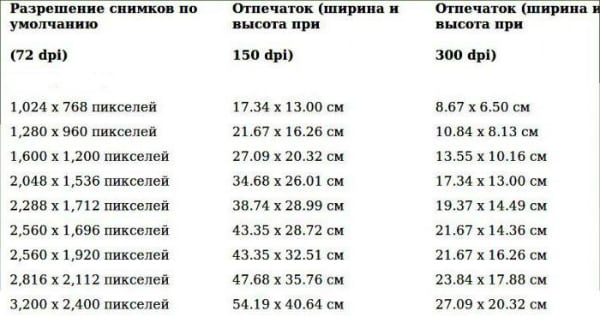Here are common screen resolutions and output sizes when printing images with these resolutions. Here the interrelation of pixel size, DPI and the physical size of the screen / matrix is ​​clearly seen.

## 3 by 4 cm how many pixels?

When printing photos, different sizes are used, 3 x 4 cm - one of them. Let's try to determine the resolution of such a photo in pixels when printing it (the printer resolution is 300 dpi). To do this, use the above formula:

x = (2.54 * p) / 300, hence

p1 = (300 * 2.3) /2.54 = 271 - the pixel width of the photo;

p2 = (300 * 4) /2.54 = 472 - pixel length;

Thus, this photo will contain 271 * 472 = 127912 pixels .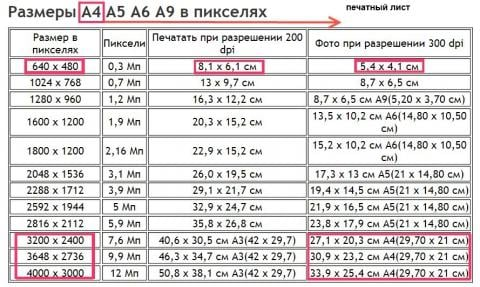Another table of the ratio of pixel resolution, sheet size and the physical size of the image at the output.

## Conclusion

With the advent of varieties of resolutions, points, drops, and so on, confusion has arisen in determining pixel density, photo size, etc. But the formulas in this article are relevant.

If you want to know more information about DPI and PPI, I suggest you look at the videos that reveal the content of these concepts:

﻿
﻿
How do you rate the article?( 2 ratings, average: 5.00 out of 5)Loading...